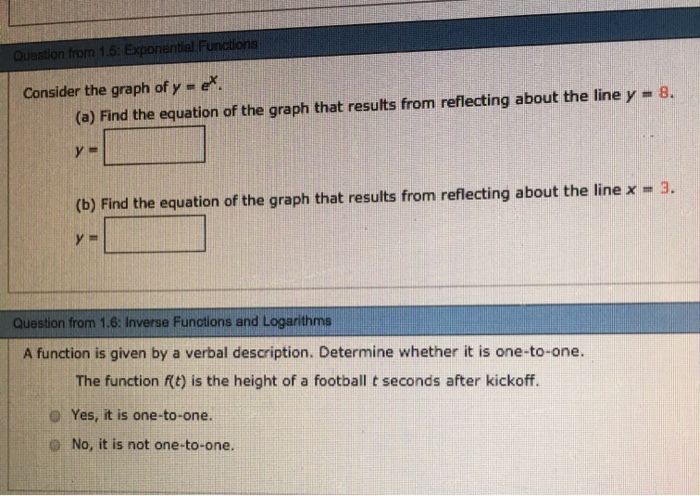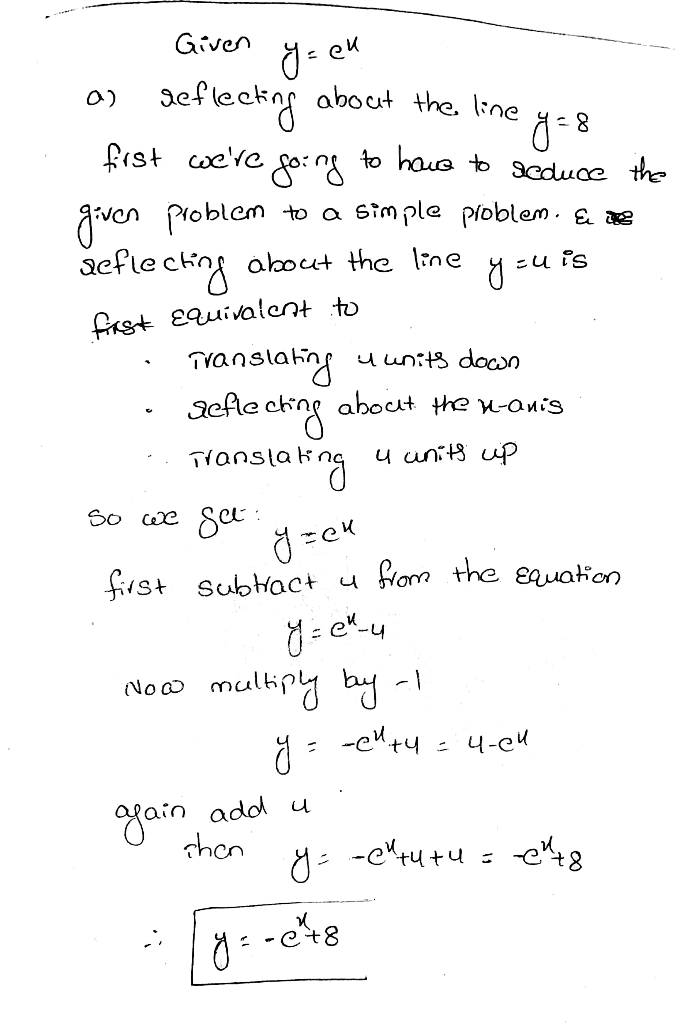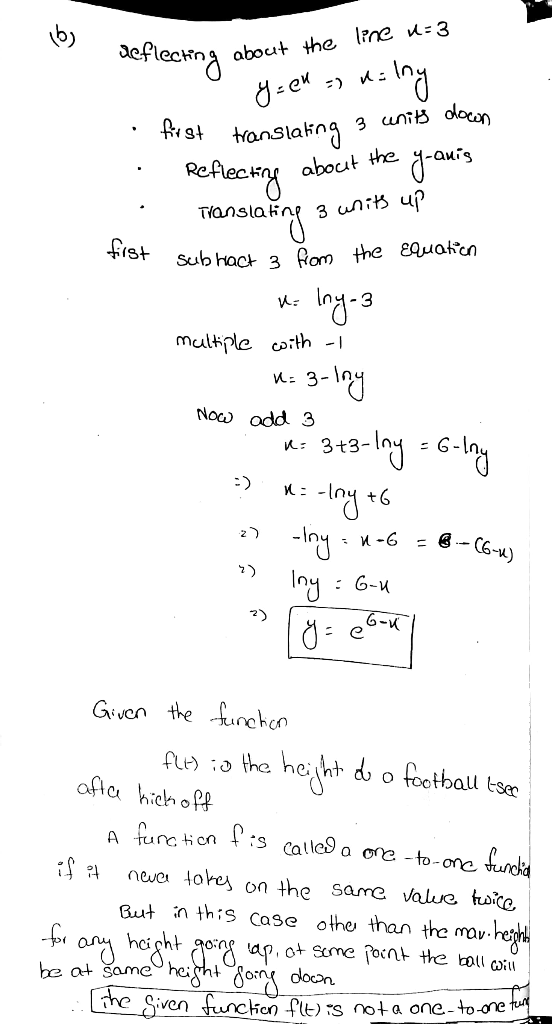# Consider the graph of y m e. 8. (a) Find the equation of the graph that results from reflecting about the line y (b...Consider the graph of y m e. 8. (a) Find the equation of the graph that results from reflecting about the line y (b) Find the equation of the graph that results from reflecting about the line x -3. Question from 1.6: Inverse Functions and Logarithms A function is given by a verbal description. Determine whether it is one-to-one. The function f(t) is the height of a football t seconds after kickoff. D Yes, it is one-to-one. O No, it is not one-to-one##### Add Answer of: Consider the graph of y m e. 8. (a) Find the equation of the graph that results from reflecting about the line y (b...
More Homework Help Questions Additional questions in this topic.